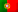Home > Technology > Computer Science > Computer Architecture > Binary-Decimal conversion with floating point

# Binary-Decimal conversion with floating point

We have learned in the previous post how to convert a number between numeral systems but for integer values. However, if we want to convert a floating point number between binary and decimal, we need more things to take into account.

In this post I’m going to explain these cases just for binary and decimal. Let’s start with another basic example.

Let’s consider the following number:

0.145

So we have one tenth (weight of 0.1), 4 hundredths (weight of 0.01) e 5 thousandths (weight of 0.001).

Representing this in powers of 10, we will have: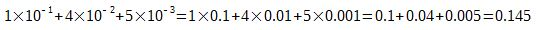## Converting from binary to decimal with floating point

Let’s now consider the following binary number:

0.01

If we used positive powers of 2 for the integer part, for the decimal places we will use negative powers of 2. In this case, we will have:This is: 0.01 (2) = 0.25 (10)

Let’s now take a look on this more complete binary number and let’s use again our frindly table:

101.11

22 = 421 = 220 = 12-1 = 0,502-2 = 0,25
101,11

We will then have: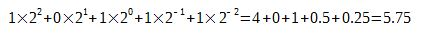This is: 101.11 (2) = 5.75 (10)

But if we now consider the fact that we have a limit given by the precision we can have with a determined number of bits, things could became a little harder. Let’s consider a limit given by 7 bits of precision and the following binary number (notice that we will write all the 7 bits even we have zeros on the right):

0.1100100

Performing the calculations: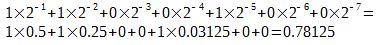Is this result final? The answer relies on an issue that exists even in the digital to analogue converters. It’s the resolution or precision! A binary number is limited to a determined number of possible combinations and this issue also applies to floating point numbers.

When we worked with integer numbers, we learned that with 7 bits we can have 27 = 128 combinations. The same thing applies to the decimal places! We can only have 128 combinations after the floating point and they are not that linear as for the integer part, as we can check:

0.10 = 0.5

0.01 = 0.25

0.11 = 0.75

This means we have some gaps but this does not mean we can’t represent 0.1 or 0.7 since these numbers might appear with more precision bits as we will see next. Now for the previous number, the problem resides on knowing if we can represent the entire number of 0.78125 with a precision of 7 bits.

In order to ensure the decimal result is in accordance with the resolution, or precision, we have with 7 bits, we have the following general condition:

10p ≤ 2n, having on “p” the number of decimal places (precision) and “n” with the number of bits. In this case, we know we have 7 bits and the condition is given by: 10p ≤ 27.

To get “p” we have to make a more complex calculation since we will need to perform a calculation with the following common logarithm: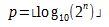Where ⌊x⌋ represents the “floor” function, that returns the highest integer number less or equal to x.

For our case, as we have 7 bits: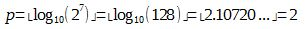This is, we just have two precision digits!

So, we can conclude that, for a 7 bit resolution, our result will be given by:

0.1100100 (2) = 0.78 (10)

To get a better understanding on this subject, let’s see if we had precisions of 10 and 16 bits…

With 10 bits, our binary number would be written like this:

0.110010000

In this case we would have: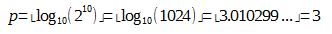And the solution given by:

0.1100100000 (2) = 0.781 (10)

Finally, with 16 bits, our binary number would be written like this:

0.110010000000000

In this case we would have:Rounding the number, the solution is:

0.1100100000000000 (2) = 0.7813 (10)

## Converting from decimal to binary with floating point

Now, let’s see how we can convert a floating point number from decimal to binary.

To convert an integer number we used successive divisions by 2. Now, for decimal places, we will successively multiply our number by 2, with a specific rule, until we reach an exact integer result of 1.

Let’s get one of the previous results in decimal:

0.25

And let’s use this table for now.

 0,25 x 2 = 0 ,50 0,50 x 2 = 1 ,00

We multiply 0.25 by 2 and we get 0.50. The final result is not 1 yet, so we multiply 0.50 by 2 and we finally get 1 (1.00).

Now, from top to bottom, we join all integer results and we get:

0.25 (10) = 0.01 (2)

This was a simple example, let’s now consider the following number:

0.75

It seems to be easy too… Let’s now carefully make the calculations step-by-step:

0.75 x 2 = 1.50

Well, we got a result greater than 1 and not 1.00! If we multiply it by 2 we will get 3 and 3 does not exist in binary! So, what could we do?

It’s easy! Let’s get the decimal places and continue with the integer part with zero!

0.50 x 2= 1.00

Finally we reached 1.00! Let’s join again all the integers from top to bottom and we get the following result:

0.75 (10) = 0.11 (2)

And this is the rule for this calculations.

Let’s see another already known decimal number:

0.78125

Let’s perform the same operations:

0.78125 x 2 = 1.5625

0.5625 x 2 = 1.125

0.125 x 2 = 0.25

0.25 x 2 = 0.5

0.5 x 2 = 1

And so we get again our binary number 0.11001.

In this case, we did not mention the precision, so that’s how we will represent it. But if we assume we have 8 precision bits for the decimal places, we will have:

0.78125 (10) = 0.11001000 (2)

And if we have less precision bits? For example, 4? Then we will have to truncate the result to 0.1100.

The resolution or precision issue can be verified in more complex numbers. The following decimal number might seem to be easy to convert, but let’s see:

0.32

Applying the rules:

0.32 x 2 = 0.64

0.64 x 2 = 1.28

0.28 x 2 = 0.56

0.56 x 2 = 1.12

0.12 x 2 = 0.24

0.24 x 2 = 0.48

0.48 x 2 = 0.96

0.96 x 2 = 1.92

When do we stop? That depends on the precision we want for the decimal places!

Let’s now see a number with both integer and decimal places! For instance:

58.125

In this case, we have to handle each one of the parts!

Let’s start with the integer 58, applying the successive divisions by 2: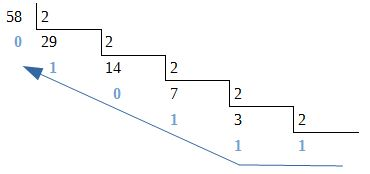We get that 58 is 111010 in bae-2.

And now the decimal places:

0.125 x 2 = 0.25

0.25 x 2 = 0.50

0.50 x 2 = 1.00

We get that 0.125 is 0,001 in binary.

Now let’s just gather everything to finally get:

58.125 (10) = 111010.001 (2)